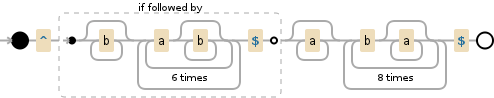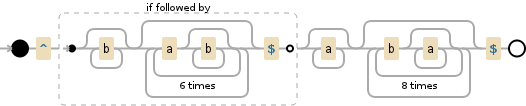# RE: Number of a's is divisible by 6 and Number of b's is divisible by 8

Find a regular expression which represents strings made of {`a,` `b`}, where number of `a`'s is divisible by 6 and number of `b`'s is divisible by `8`.

I tried to create a DFA which accepts such strings. My idea was to use all the remainders mod 6 and mod 8 leading to a total of 48 remainders. Thus each state in DFA is a pair `(r, s)` where `r` varies from 0 to 6 and `s` varies from 0 to 7. Start state (as well as accepting state) is `(0, 0)` and by we can easily give transitions to states by noting that if we input `"a"` the state `(r, s)` transitions to `(r + 1, s)` and on input `"b"` it transitions to state `(r, s + 1)`.

However it is too difficult to work with a DFA of 48 states and I am not sure if this can be minimized by using the DFA minimization algorithm by hand.

I am really not sure how then we can get to a regular expression representing such strings.

• No this can't minimized and its regular expression would be very long and complex, even if you try to find regex for 'b' /2 and 'a' / 2 will be very complex Read this – Grijesh Chauhan Apr 18 '14 at 12:24
• From the final regular expression I have written you should understood that you need to write all possible combination for 6a and 8b -- Yes you can write comparatively simple "Regex" (not regular expression in formal languages). – Grijesh Chauhan Apr 18 '14 at 12:28
• Your proposed DFA is already minimal. – Willem Van Onsem Apr 18 '14 at 12:38
• Thanks to all for the comments! I was actually trying to teach this DFA / regex topic to someone and found that they got this question in exam. I think the question should have been limited to the description of DFA rather than finding an actual regex. – Paramanand Singh Apr 18 '14 at 13:03
• If you need more appropriate answer read this Minimum number of states in DFA for the given language? – Grijesh Chauhan Apr 18 '14 at 13:39

If you are allowed to use lookaheads:

``````^(?=b*((ab*){6})+\$)a*((ba*){8})+\$
``````Debuggex Demo

Example of matched string: `bbaabbaabbaabb`

Idea is simple: We know how to match string having number of `a`s divisible by 6 - `^((b*ab*){6})+\$`, also we know how to match string having number of `b`s divisible by 8 - `^((a*ba*){8})+\$`. So we just put one regex to lookahead, and another one to matching part.

In case if you also need to match strings consisting only of `a`s or only of `b`s, then the following regex will do:

``````^(?=b*((ab*){6})*\$)a*((ba*){8})*\$
``````Examples of matched strings: `aaaaaa`, `bbbbbbbb`, `bbaabbaabbaabb`

Debuggex Demo

• Can you share any word matching your expression and condition. Unfortunately I am not able to match to required word. – Murthy Apr 18 '14 at 14:10
• @Murthy I added link to demo. Basically any string consisting of `a` and `b`, where number of `a`s is divisible by 6 and number of `b`s is divisible by 8 will match. – Ulugbek Umirov Apr 18 '14 at 14:13
• @ Ulugbek Umirov I tried the word "babbabbaabbaab" which is tried at online regex evaluator Please share any working word for my understanding of expression. – Murthy Apr 18 '14 at 14:19
• @Murthy OP stated `made of {a, b}`, it means only `a` and `b` symbols are allowed. – Ulugbek Umirov Apr 18 '14 at 14:20
• Thanks for sharing the link – Murthy Apr 18 '14 at 14:23